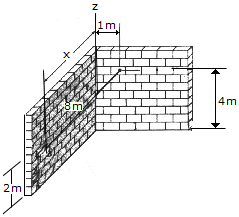# Engineering Mechanics - Force Vectors - Discussion

### Discussion :: Force Vectors - General Questions (Q.No.1)

1.The cord is attached between two walls. If it is 8 m long, determine the distance x to the point of attachment at B.

 [A]. x = 7.75 m [B]. x = 7.68 m [C]. x = 6.93 m [D]. x = 7.94 m

Explanation:

No answer description available for this question.

 D.Vengadesan said: (Dec 15, 2010) Sir I want explanation for this answer.

 Jay Aswani said: (Jan 4, 2012) Sir please provide complete solution so that we can take advantage of that.

 Mr. Right said: (Sep 9, 2013) The answer is actually 7.68. The diagram is not a right triangle, as it is in 3 dimensions and not on an x,y axis. Correct approach: A=0i+1j+4z. B=?i+0j+2z. Distance between 2 points in 3d: sqrt((x2-x1)^2+(y2-y1)^2+(z2-z1)^2). (x-0)^2 + (0-1)^2 + (2-4)^2. x^2 + 1 + 4 = 8^2. x^2=59. x= sqrt59 = 7.68115. Now if you check that you will see that it is correct, as opposed to the answer that you all tried to simplify and didn't bother to check your work.

 Second Right said: (Jul 26, 2014) I agree with Mr. Right, there are two perpendicular right triangles that you must solve. This is an engineering mechanics question, not a grade 10 trigonometry question. The answer is x=sqrt(59).

 Riyaz Ibrahim said: (Oct 5, 2015) I am not satisfied, try to solve in simple format.

 Woxiiii said: (Nov 22, 2015) It's 3D Pythagoras so all you have to do is substitute into the formula a^2+b^2+c^2=d^2.

 Abdul Khaleqaue said: (Dec 22, 2015) Suppose B point at black wall and A point at white wall. Draw a line perpendicular to white wall. Now apply Pythagoras formula. You will find square root of (8^2-(4-2)^2) = 7.7459. It will give you hypotenuse of new triangle. Again apply same formula square root of (7.7459^2-1^2) = 7.68.

 Karthi said: (Dec 28, 2015) Sir I cannot understand so please explain.

 Nicky said: (Feb 17, 2016) Please explain it into details.

 Susheel said: (Feb 29, 2016) More explain.

 Alex said: (May 2, 2017) Please, can anyone make it clear?

 Prahlad said: (Oct 3, 2017) 2^2-8^2-1^2=59 , Then √59=7.68.

 Kunal said: (Jan 19, 2019) 8^2=1^2+2^2+x^2. x^2=59, x = 7.68,